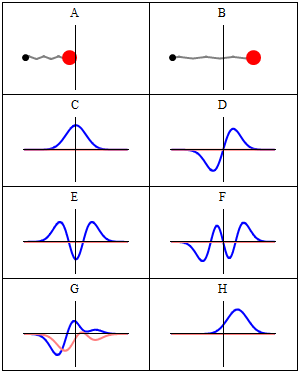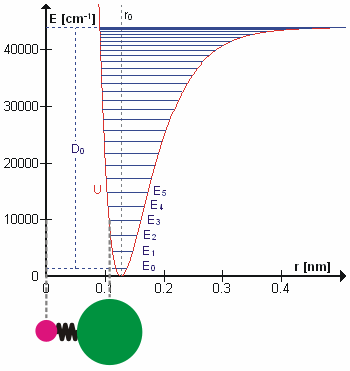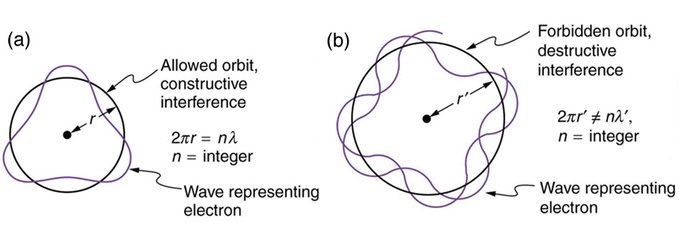Lecture 13: Harmonic Oscillators and Rotation of Diatomic Molecules

•• Contributed by Delmar Larsen
• Founder and Director at Libretexts

Recap of Lecture 12

Last lecture laid the groundwork for understanding spectroscopy. We first introduce bra-key notation as a means to simplify the manipulation of integrals. We introduced a qualitative discussion of IR spectroscopy and then focused on "selection rules" for what vibrations are "IR-active" and can be seen in IR spectra. The two criteria we got were that the vibration requires a changing dipole moment (a static dipole is not needed as discussed for $$CO_2$$) and that $$\Delta v = \pm 1$$ for the transition. We were setting the ground work for explaining how to derive the second selection rule using the concept of a transition moment and symmetry. We will pick up that discussion there.

More on Dirac's Bra-Ket notation

In the early days of quantum theory, P. A. M. (Paul Adrian Maurice) Dirac created a powerful and concise formalism for it which is now referred to as Dirac notation or bra-ket (bracket $$\langle \, | \, \rangle$$) notation. Bra-ket notation is a standard notation for describing quantum states in the theory of quantum mechanics composed of angle brackets and vertical bars. It can also be used to denote abstract vectors and linear functionals in mathematics.

• kets: $$| \, \rangle$$
• bras: $$\langle \, |$$
• Bra-Ket Pairs (dot products): $$\langle Φ|Ψ \rangle$$, consisting of a left part, $$\langle Φ|$$, (the bra), and a right part, $$|Ψ\rangle$$, (the ket).

Example

For the ground state of the well-known particle-in-a-box of unit dimension.

$\langle x | \Psi \rangle = \Psi(x) \ 2^{1/2} \sin (\pi x)$

However, if we wish to express $$\Psi$$ in momentum space we would write

$\langle p | \Psi \rangle = \Psi(p) = 2^{1/2} \dfrac{e^{-ip} +1}{\pi^2 - p^2}$

How one finds this latter expression will be discussed later.

We ended class on Wednesday discussing the symmetries of productions of functions that have symmetries.

Product table Odd Function (anti-symmetric) Even Function (symmetric) No symmetry (neither odd nor even)
Odd Function (anti-symmetric) Even Function (symmetric) Odd Function (anti-symmetric) who knows
Even Function (symmetric) Odd Function (anti-symmetric) Even Function (symmetric) who knows
No symmetry (neither odd nor even) who knows who knows who knows

And now the Harmonic Oscillator Wavefunctions

Because of the association of the wavefunction with a probability density, it is necessary for the wavefunction to include a normalization constant, $$N_v$$.

$N_v = \dfrac {1}{(2^v v! \sqrt {\pi} )^{1/2}} \label {5.6.15}$

The final form of the harmonic oscillator wavefunctions is the product of thee terms:

$\color{red} | \Psi _v (x) \rangle = N_v H_v (x) e^{-\dfrac {x^2}{2}} \label {5.6.16}$

The first few physicists' Hermite polynomials are:

$$H_0(x)=1\,$$

$$H_1(x)=2x\,$$

$$H_2(x)=4x^2-2\,$$

$$H_3(x)=8x^3-12x\,$$

$$H_4(x)=16x^4-48x^2+12\,$$

$$H_5(x)=32x^5-160x^3+120x\,$$

The symmetry of the Harmonic Oscillator wavefunctions is dictated by the Hermite Polynomials• Which levels are even functions and which are odd functions?
• Without calculating an integral, what is $$\langle x\rangle$$?

Full time dependence of the Wavefunctions

The wavefunctions in Equation \ref{5.6.16} are the time-independent parts to the total wavefunction. As discussed previously, the total time-dependent wavefunctions can be expressed (if no time dependent potential is used as)

$| \Psi_v(x,t) \rangle = | \psi_v(x) \rangle e^{-iEt/\hbar}$

Where $$v$$ is the Harmonic oscillator quantum number (i.e. how excited the vibration is). This means that there is both a real and imaginary component to the wavefunction as it oscillates in time.

$| \Psi_v(x,t) \rangle = | \psi_v(x) \rangle \cos \left(Et/\hbar\right) - i | \psi_v(x) \rangle \sin \left(Et/\hbar \right)$

This is visualized below.A harmonic oscillator in classical mechanics (A-B) and quantum mechanics (C-H). In (A-B), a ball, attached to a spring (gray line), oscillates back and forth. In (C-H), wavefunction solutions to the Time-Dependent Schrödinger Equation are shown for the same potential. The horizontal axis is position, the vertical axis is the real part (blue) or imaginary part (red) of the wavefunction. (C,D,E,F) are stationary states (energy eigenstates), which come from solutions to the Time-Independent Schrodinger Equation. (G-H) are non-stationary states, solutions to the Time-Dependent but not Time-Independent Schrödinger Equation. (G) is a randomly-generated superposition of the four states (E-F). H is a "coherent state" ("Glauber state") which somewhat resembles the classical state B. Image used with permission (Sbyrnes321)

When can a vibration transition be IR active (within HO approximation)?

This is done via the transition moment integral $$\langle \psi_i | \hat{M}| \psi_f \rangle$$. The transition moment integral gives information about the probability of a transition occurring. For IR of a single harmonic oscillator, $$\hat{M}$$ can be set to $$x$$. A more detailed discussion will be presented later. So the probability for a transiton in HO is $P_{i \rightarrow f} = \langle \psi_i | x | \psi_f \rangle$

• When the vibration has a changing dipole moment
• When the adjacent eigenstates $$v'=v\pm 1$$ are involved (no harmonics or overtones)

However overtones are actually experimentally observed

Overtones occur when a vibrational mode is excited from $$\nu=0$$ to $$\nu=2$$, which is called the first overtone, or $$\nu=0$$ to $$\nu=3$$, the second overtone. The fundamental transitions, v=±1, are the most commonly occurring, and the probability of overtones rapid decreases as $$\nu=\pm n$$ increases. Based on the harmonic oscillator approximation, the energy of the overtone transition will be about n times the fundamental associated with that particular transition. The anharmonic oscillator calculations show that the overtones are usually less than a multiple of the fundamental frequency. This is demonstrated with the vibrations of the diatomic HCl in the gas phase:

Table 1: HCl vibrational spectrum.
Transition obs [cm-1] obs Harmonic [cm-1] obs Anharmonic [cm-1]
$$0 \rightarrow 1$$ (fundamental) 2,885.9 2,885.9 2,885.3
$$0 \rightarrow 2$$ (first overtone) 5,668.0 5,771.8 5,665.0
$$0 \rightarrow 3$$ (second overtone) 8,347.0 8,657.7 8,339.0
$$0 \rightarrow 4$$ (thirdovertone) 10,923.1 11,543.6 10,907.4
$$0 \rightarrow 5$$ (fourth overtone) 13,396.5 14,429.5 13,370

We can see from Table 1, that the anharmonic frequencies correspond much better with the observed frequencies, especially as the vibrational levels increase.

The Harmonic Oscillator is just an approximation of the True PotentialThe HCl molecule is really an anharmonic oscillator vibrating at energy level $$E_3$$. $$D_0$$ is dissociation energy here, $$r_0$$ bond length, $$U$$ potential energy. Energy is expressed in wavenumbers. The hydrogen chloride molecule is attached to the coordinate system to show bond length changes on the curve. Image used with permission from Wikipedia.

The Taylor series for an arbitrary potential energy curve $$V(x)$$ is defined by:

$V(x) = U(x_0) + (x-x_0) \left.\dfrac{dV}{dx}\right|_{x_0} + \dfrac{(x-x_0)^2}{2!} \left.\dfrac{d^2V}{dx^2}\right|_{x_0} + \cdots \label{tay1}$

The system will tend to settle into the configuration where $$U(x)$$ has a minimum --- but, by definition, that's where the first derivative $$dV/dx=0$$ vanishes. Also a constant offset to a potential energy usually does not affect the physics. That leaves us with

$V(x) = \dfrac{(x-x_0)^2}{2!} \left.\dfrac{d^2V}{dx^2}\right|_{x_0} + \mathcal O(x-x_0)^3 \approx \dfrac12 k (x-x_0)^2 \label{tay2}$

which is the harmonic oscillator potential for small oscillations around $$x_o$$.Image of potential of a true molecular vibration and the harmonic oscillator approximation. Image used with permission from Wikipedia.

If the cubic term is not ignored, then the expansion can be rewritten as (taking the first two important terms of Equation $$\ref{tay1}$$)

$V(\ell) = \dfrac {1}{2} kx^2 + \dfrac {1}{6} \gamma x^3 \label{tay3}$

where

• $$V(\ell_0) = 0$$, and $$x = \ell - \ell_0$$.
• $$k$$ is the harmonic force constant, and
• $$\gamma$$ is the anharmonic term.

$$V(\ell)$$ is typically shortened to the cubic term (but not always). It is important to note that this approximation is only good for $$\ell$$ near $$\ell_0$$, and that $$\ell_0$$ stands for the equilibrium bond distance. Almost all diatomics have experimentally determined $$\dfrac {d^2 V}{d \ell^2}$$ for their lowest energy states. H2, Li2, O2, N2, and F2 have had terms up to $$n < 10$$ determined.

The Morse Potential (A better potential for describing Vibrations than Harmonic Oscillator Potential)

The Morse potential, named after physicist Philip M. Morse, is a convenient interatomic interaction model for the potential energy of a diatomic molecule. It is a better approximation for the vibrational structure of the molecule than the quantum harmonic oscillator because it explicitly includes the effects of bond breaking, such as the existence of unbound states. It also accounts for the anharmonicity of real bonds and the non-zero transition probability for overtone and combination bands.

The Morse Potential is a good approximation to $$V(\ell)$$ and is best when looking for a general formula for all $$\ell$$ from 0 to $$\infty$$, not just applicable for the local region around the $$\ell_o$$:

$V(\ell) = D(1-e^{-\beta (\ell - \ell_0)})^2 \label{2}$

with

• $$V(\ell = \ell_0) = 0$$ and
• $$V(\ell = \infty) = D$$Morse Potential more closely resembles the true vibration potential than a harmonic oscillator. Image used with permission (CC BY-SA 3.0; Mark Somoza)

For H2, the equilibrium bond length $$\ell_0 = 74.1 pm$$, the bond energy $$D = 7.61 \times 10^{-19} J$$, and the Morse potential parameter $$\beta = 0.0193 pm^{-1}$$.

To solve for energy using the relevant formulas $$\hat{H} \psi = E\psi$$ and $$E = K + V$$, an approximation must be made, saying that $$V(x) = \dfrac {1}{2} kx^2$$. The kinetic energy is known because the motion is one dimensional, so the kinetic energy operator $$\hat{K}$$ in the Particle in the Box problem can be used. The equation $$\hat{H} \psi = E\psi$$ now becomes

$\hat{H} \psi = \dfrac {\hbar}{2\mu} \dfrac {d^2 \psi}{d x^2} + \dfrac {1}{2}kx^2 = E\psi \label{3}$.

Note that $$\mu$$ is used as the reduced mass, not $$m$$. For a diatomic molecule,

$\mu = \dfrac {m_1 m_2}{m_1 + m_2}$

Solving for the energy gives quantized results in terms of $$v$$ - $$E_v = \hbar (\sqrt {\dfrac {k}{\mu}}) (v+ \dfrac {1}{2})$$, which can be simplified to $$E_v = h\tilde{v} (v+\dfrac {1}{2})$$, having $$cm^{-1}$$ units.

Rotations

Classical Rotation of a Diatomic Molecule

Angular momentum can be considered a rotational analog of linear momentum. Thus, where linear momentum is proportional to mass $$m$$ and linear speed $$v$$.

$p =mv \tag{linear momentum}$

angular momentum $$J$$ is proportional to moment of inertia $$I$$ and angular speed $$\omega$$.

The moment of inertia $$I$$ of a rigid body determines the torque needed for a desired angular acceleration about a rotational axis.For a diatomic

$I= \mu r^2$

• where $$\mu$$ is the reduced mass discussed previously $\mu= \dfrac{m_1m_2}{m_1+m_2} \tag{reduced mass}$
• $$r$$ is the bond length (remember no vibration occurs in rigid rotors, so this is the equilibrium bond length)

The angular momentum perpendicular to the rotational motion $$L_z$$ is

$L_z = mvr \tag{angular momentum}$

with energies

$E=\dfrac{L_z^2}{2I} \tag{classical energy of a rigid rotor}$The angular velocity describes the speed of rotation and the orientation of the instantaneous axis about which the rotation occurs. The direction of the angular velocity pseudovector will be along the axis of rotation; in this case (counter-clockwise rotation) the vector points up. Image used with permission from Wikipedia

2D Quantum Rigid Rotor (rotation in a single plane)

The angular momentum of a rigid rotor is

$L_z = pr$

Using the de Broglie relation $$p = \dfrac{h}{\lambda}$$ we also have a condition for quantization of angular motion

$L_z= \dfrac{hr}{\lambda}$

The wavelength must be a whole number fraction of the circumference for the ends to match after each circuit (remember the Bohr atom discussion).(A) Waves on a string have a wavelength related to the length of the string, allowing them to interfere constructively. (B) If we imagine the string bent into a closed circle, we get a rough idea of how electrons in circular orbits can interfere constructively. (c) If the wavelength does not fit into the circumference, the electron interferes destructively; it cannot exist in such an orbit. Image used with permission from Boundless.

Hence, the condition

$\dfrac{2\pi r}{m} =\lambda$

combined with the deBroglie relation leads to a quantized expression of angular momentum

$L_z=m\hbar \tag{quantization of angular momentum}$

The Hamiltonian is for a rigid rotor is then

$- \dfrac{\hbar^2}{2I} \dfrac{\partial ^2 }{\partial \phi^2} \tag{RR Hamiltonian}$

The corresponding wavefunctions are:

$|\Phi_m \rangle = \sqrt{\dfrac{1}{2\pi}} e ^{im\phi} \tag{eigenstates of a 2D RR}$

with the constraint that:

$m =\pm \dfrac{\sqrt{2IE}}{\hbar} \tag{eigenenergies of a 2D RR}$

Since the energy is constrained to values

$E = \dfrac{L_z^2}{2I} \tag{Energies are quantized}$

we find that the quantum numbers fo rotation are

$m = 0, \pm 1,\pm, \pm 2, ... \tag{quantum number for 2D RR}$

Properties and Notes about 2D rigid Rotor

• Solutions of the 2D Rigid Rotor rotational Hamiltonian are sine and cosine functions just like the particle in a box.
• Here the boundary condition is imposed by the circle and the fact that the wavefunction must not interfere with itself.
• This model is similar to condition in the Bohr model of the atom
• The energy and wavefunctions are dependent on the quantum number $$m$$ that varies from $$0$$ to $$\infty$$.